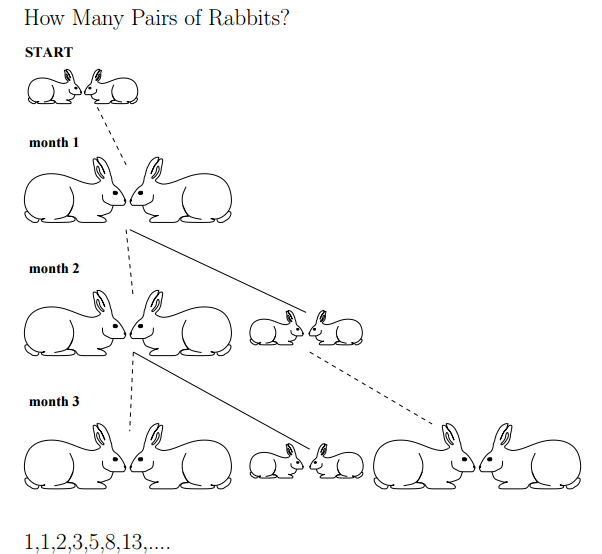# LEARN FIBONACCI NUMBERS#DEFINE FIBONACCI NUMBERS

An online fibonacci number definition

Leonardo Fibonacci, originally known as Leonardo of Pisa, was an Italian merchant and mathematician who contributed much to the field of algebra, Euclidian geometry, Diophantine equations, and number theory. Among his many writings was the Liber Abaci, published in 1202, which contained a famous problem about rabbits which led to what we refer to today as Fibonacci numbers or the Fibonacci sequence

The famous rabbit problem has been quoted many ways in historical literature but basically asks, Start with a single pair of rabbits. Any pair of rabbits of one generation will produce a pair for the next generation, and then another pair of rabbits for the generation after that. But then they will die. How many rabbits will be produced in the nth generation?

The answer to this well-known problem is the famed Fibonacci sequence: 1, 1, 2, 3, 5, 8, 13, 21 and so on to get each subsequent term, add the sum of the two numbers that precede it. For example, 1+1=2= the third term in the sequence.Here is a other example to calculate the sum of the first 12 even Fibonacci numbers.

## Sum Of First 12 Even Fibonacci Numbers

Recall that the Fibonacci sequence is a series that begins with two integers: 0, 1. The next number in the sequence is derived by adding the previous two numbers, so the Fibonacci sequence looks like this: 0, 1,1, 2, 3, 5, 8, 13, 21, 34, ...

Click the button to see the results of "sum of the first 12 even Fibonacci numbers"

The sum of the first 12 even Fibonacci numbers is:#Related Number Types

##Math number calculation ►

Online Algebra calculation, formulas , Digital calculation, Statistical calculation, Math Converters Pet Age Calculator